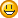SEARCH HOMEMath Central Quandaries & QueriesQuestion from hector, a student: how can i find out how much water i can fill my bedroom with when it measures 12 ft.x12 ft.x10 ft.We have two solutions for you

Hector,

To find the volume in cubic feet multiply the length tims the width times the height. There are 7.48 US gallons in a cubic foot so if you want the volume in gallons multiply the volume in cubic feet by 7.48.

Penny

Hector,

If your walls are strong enough, then they can contain water filling the volume 12 x 12 x 10 = 1440 cubic feet.

Since 1 cubic foot of water is equal to 28.3 liters, this is 1440 x 28.3 = about 40 750 liters

Um....don't try this at home.Cheers,
Stephen La Rocque.Math Central is supported by the University of Regina and The Pacific Institute for the Mathematical Sciences.